# PSAT Math : Geometry

## Example Questions

### Example Question #1 : Squares

If the area of a square isunits squared, what is the length of its diagonal?units

Not Enough Informationunits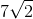units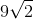unitsunits

Explanation:

The diagonal of a square creates two special 45-45-90 triangles, meaning that the diagonal of a square is just the length of one side of the square multiplied by the square root of 2.

In this problem, you can figure out the length of one side of the square by finding the square root of the area (which is equal to a side length), then multiplying that number by the square root of 2.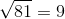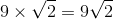### Example Question #1 : How To Find The Area Of A Square

ABCD and EFGH are squares such that the perimeter of ABCD is 3 times that of EFGH. If the area of EFGH is 25, what is the area of ABCD?

25

15

225

5

75

225

Explanation:

Assign variables such that

One side of ABCD = a

and One side of EFGH = e

Note that all sides are the same in a square. Since the perimeter is the sum of all sides, according to the question:

4a = 3 x 4e = 12e or a = 3e

From that area of EFGH is 25,

e x e = 25 so e = 5

Substitute a = 3e so a = 15

We aren’t done. Since we were asked for the area of ABCD, this is a x a = 225.

### Example Question #1 : How To Find The Area Of A Square

A square has an area of 36. If all sides are doubled in value, what is the new area?

132

72

144

108

48

144

Explanation:

Let S be the original side length. S*S would represent the original area. Doubling the side length would give you 2S*2S, simplifying to 4*(S*S), giving a new area of 4x the original, or 144.

### Example Question #231 : Geometry

If the perimeter of a square is equal to twice its area, what is the length of one of its sides?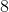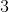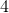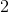Explanation:

Area of a square in terms of each of its sides:

Area = S x S

Perimeter of a square:

Perimeter = 4S

So if 'the perimeter of a square is equal to twice its area':

2 x Area = Perimeter

2 x [S x S] = [4S]; divide by 2:

S x S = 2S; divide by S:

S = 2

### Example Question #2 : Squares

Freddie is building a square pen for his pig. He plans to buy x feet of fencing to build the pen. This will result in a pen with an area of p square feet. Unfortunately, he only has enough money to buy one third of the planned amount of fencing. Which expression represents the area of the pen he can build with this limited amount of fencing?

p/6

p/9

3p

p/3

9p

p/9

Explanation:

If Freddie uses x feet of fencing makes a square, each side must be x/4 feet long. The area of this square is (x/4)2 = x2/16 = p square feet.

If Freddie uses one third of x feet of fencing makes a square, each side must be x/12 feet long. The area of this square is (x/12)2x2/144 = 1/9(x2/16) = 1/9(p) = p/9 square feet.

Alternate method:

The scale factor between the small perimeter and the larger perimeter = 1 : 3. Since we're comparing area, a two-dimensional measurement, we can square the scale factor and see that the ratio of the areas is 1: 32 = 1 : 9.

### Example Question #81 : Quadrilaterals

If the diagonal of a square measures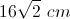, what is the area of the square?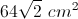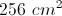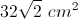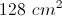Explanation:

This is an isosceles right triangle, so the diagonal must equal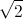times the length of a side. Thus, one side of the square measures, and the area is equal to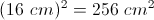### Example Question #1 : Squares

A square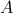has side lengths of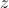. A second square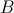has side lengths of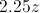. How many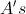can you fit in a single?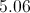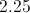Explanation:

The area ofis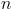, the area ofis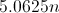. Therefore, you can fit 5.06in.

### Example Question #91 : Quadrilaterals

The perimeter of a square is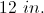If the square is enlarged by a factor of three, what is the new area?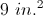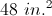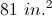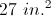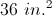Explanation:

The perimeter of a square is given by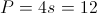so the side length of the original square is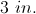The side of the new square is enlarged by a factor of 3 to give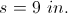So the area of the new square is given by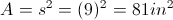.

### Example Question #12 : How To Find The Area Of A Square

A half circle has an area of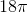. What is the area of a square with sides that measure the same length as the diameter of the half circle?

81

144

36

72

108

144

Explanation:

If the area of the half circle is, then the area of a full circle is twice that, or.

Use the formula for the area of a circle to solve for the radius:

36π = πr2

r = 6

If the radius is 6, then the diameter is 12. We know that the sides of the square are the same length as the diameter, so each side has length 12.

Therefore the area of the square is 12 x 12 = 144.

### Example Question #1 : How To Find The Length Of The Side Of A Square

The area of square R is 12 times the area of square T. If the area of square R is 48, what is the length of one side of square T?

2

1

16

4

2

Explanation:

We start by dividing the area of square R (48) by 12, to come up with the area of square T, 4.  Then take the square root of the area to get the length of one side, giving us 2.

### All PSAT Math Resources